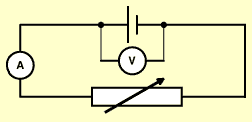## 2009-02-08

### Finding the e.m.f. and internal resistance of a cell

Usually we can find the e.m.f and internal resistance of a cell by means of graphical method.

The basic procedures are explained below:To find the e.m.f. and internal resistance of a cell the circuit above is set up.

The variable resistor is altered and readings of potential differences across the terminals of the cell and current through the cell are taken. A graph of these results is then drawn .At the point at which the line meets the terminal potential difference axis there is no current drawn from the cell. (y intercept). This is called the open circuit potential of the circuit. Because there is no current there will be no lost volts so this value is the e.m.f. of the cell. Where the line meets the terminal potential difference axis is the e.m.f. of the cell.

To find the internal resistance of the cell the gradient of the line is calculated. This has a negative value. The internal resistance of the cell is the same value but without the negative sign. For example, if the slope of the line is -4 then the internal resistance is 4 ohm.

Source: http://www.bbc.co.uk/scotland/education/bitesize/higher/physics/elect/energy_volts3_rev.shtml